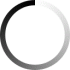Rs. 150.00Rs. 120.00
Rs. 150.00Rs. 120.00
Rs. 150.00Rs. 120.00
Rs. 150.00Rs. 120.00
Rs. 150.00Rs. 120.00
Rs. 150.00Rs. 120.00
Rs. 150.00Rs. 120.00
Rs. 150.00Rs. 120.00
Rs. 150.00Rs. 120.00
Rs. 150.00Rs. 120.00
Out of stock
Iruve in collaboration with Vasu Dixit+Gubbi is excited to be the official merchandise partner for Aagalla Anbeda. Aagalla Anbeda...
Rs. 150.00Rs. 120.00
Rs. 150.00 Rs. 120.00
Rs. 150.00 Rs. 120.00
Rs. 150.00 Rs. 120.00
Rs. 150.00 Rs. 120.00
Rs. 150.00 Rs. 120.00
Rs. 150.00 Rs. 120.00
Rs. 150.00 Rs. 120.00
Rs. 150.00 Rs. 120.00
Rs. 150.00 Rs. 120.00
Rs. 150.00 Rs. 120.00
Rs. 120.00
Rs. 150.00 Rs. 120.00